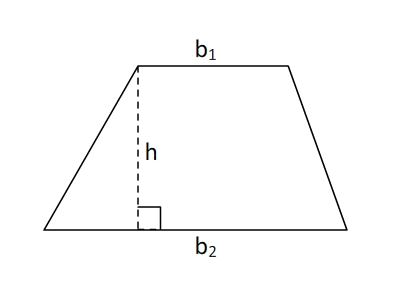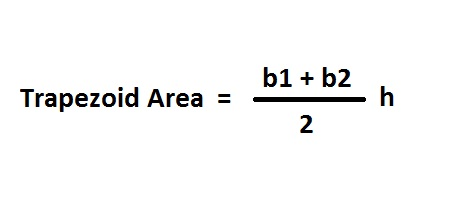# Trapezoid Area Calculator

Trapezoid Area Calculator tool is used to calculate area of a trapezoid, when the two base b1, b2 and height h is known.## Definition - What is Trapezoid?

The definition that most north American mathematicians use for trapezoid is a four-sided shape with exactly one pair of opposite sides parallel.

A trapezoid is a quadrilateral that has one pair of sides which are parallel. These two sides are called the bases of the trapezoid. The height of a trapezoid is a segment that connects the one base of the trapezoid and the other base of the trapezoid and is perpendicular to both of the bases.b1=the one base of the trapezoid

b2=the other base of the trapezoid

h=the height of the trapezoid

Altitude

An altitude of a trapezoid is a segment drawn from any point on one of the parallel sides (base) perpendicular to the opposite side (the other base). An infinite number of altitudes may be drawn in a trapezoid.

Median

A median of a trapezoid is the segment that joins the midpoints of the nonparallel sides (legs).